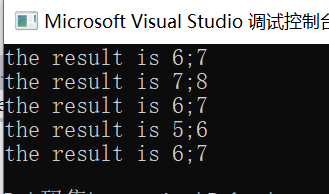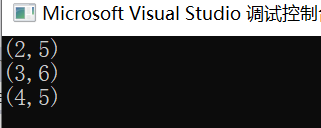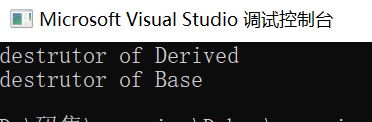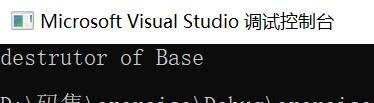# 关于多态

## 运算符重载

### 2.重载规则

a.只能重载c++已有的运算符（除了少数几个外都可以重载）。 b.重载之后运算符的优先级和结合性不变。 c.一般来说，重载的功能与原有功能类似，不能改变原运算符的操作对象个数，同时至少要有一个操作对象是自定义类型。 d.规定：类属关系运算符"."，成员指针运算符".*"，作用域分辨符"::"，三目运算符"?:"是不能重载的。 ### 3.重载的形式 #### 重载为类的非静态成员函数 ##### a.一般语法形式
返回类型 operator 运算符(形参表)
{
函数体
}


##### c.具体实现（以自增自减为例实现）
#include"pch.h"
#include<iostream>
using namespace std;
class Point//定义一个Point类
{
public:
Point(int x,int y):x(x),y(y)
{}
//前置，单目运算所以没有形参
//前置实现之后结果是左值，并且在实现的时候要实现数据共享，所以传引用
Point& operator++();
Point& operator--();

//后置，有一个int参数仅用于区分前置与后置
Point operator++(int);
Point operator--(int);
void show()
{
cout << "the result is " << x <<";"<<y<< endl;
}
private:
int x,y;
};
//类的非静态函数成员实现
Point& Point::operator++()
{
x++;
y++;
return *this;
}

Point& Point::operator--()
{
x--;
y--;
return *this;
}

Point Point::operator++(int)
{
Point old = *this;
x++;
y++;
return old;
}

Point Point::operator--(int)
{
Point old = *this;
x--;
y--;
return old;
}
int main()
{
Point po(6,7);
po++.show();//6,7
po--.show();//7,8
po.show();//6,7
(--po).show();//5,6
(++po).show();//6,7
return 0;
}#### 重载为非成员函数

##### a.一般语法形式
返回类型 operator 运算符(形参表)
{
函数体
}


##### d.具体实现（以复数类为例实现）
#include"pch.h"
#include<iostream>
using namespace std;
class Complex
{
public:
Complex(double real=0.0, double image=0.0) :real(real), image(image)
{

}
//友元函数实现
friend Complex& operator++(Complex &c1);
friend Complex operator++(Complex &c1,int);
void show()
{
cout << "(" << real << "," << image << ")" << endl;
}
private:
double real, image;
};
Complex& operator++(Complex &c1)
{
c1.real++;
c1.image++;
return c1;
}
Complex operator++(Complex &c1, int)
{
Complex c2 = c1;
++(c1);
return c2;
}
int main()
{
Complex a(3.0, 4.0);
Complex b(2.0, 5.0);
(b++).show();//2,5
b.show();//3,6
(++a).show();//4,5
return 0;
}## 虚析构函数实现多态

### 关于它的实现

#include"pch.h"
#include<iostream>
using namespace std;
class Base
{
public:
Base()
{}
virtual ~Base()//虚析构函数
{
cout << "destrutor of Base" << endl;
}
};
class Derived :public Base
{
public:
Derived()
{}
~Derived()
{
cout << "destrutor of Derived" << endl;
}
};
int main()
{
//先生成Base对象，然后生成Derived对象，返回派生类地址给基类指针
Base *p = NULL;
p = new Derived;
delete p;
return 0;
}## 纯虚函数以及抽象类

### 纯虚函数

#### 声明格式

virtual 函数类型 函数名(参数表)=0;

#### 注意点

posted on 2019-10-25 22:55  duoraemon  阅读(153)  评论(0编辑  收藏  举报